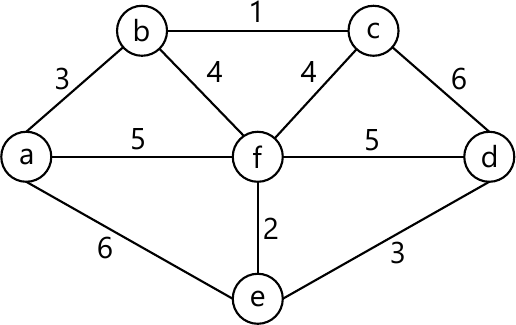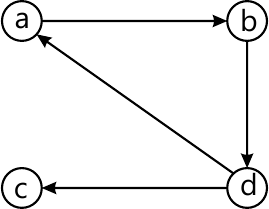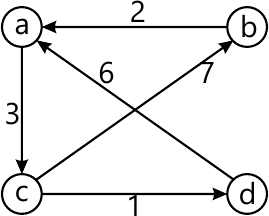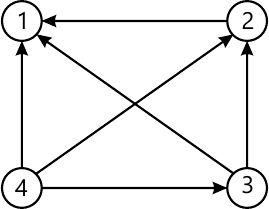MORE IN Design and Analysis of Algorithms
VTU Computer Science (Semester 4)
Design and Analysis of Algorithms
December 2013
Total marks: --
Total time: --
INSTRUCTIONS
(1) Assume appropriate data and state your reasons
(2) Marks are given to the right of every question
(3) Draw neat diagrams wherever necessary

1 (a) With the help of a flow chart. Explain the various steps of algorithm design and analysis process.
8 M
1 (b) If f1(n) ∈ O(g1(n)) and f2(n) ∈ O(g2(n)) prove that f1(n)+f2(n) ∈ O(max |g(n).g2(n)|)
4 M
1 (c) Write an algorithm for selection sort and show that the time complexity of this algorithm is quadratic.
8 M

2 (a) What is divide and conquer method. Show that the worst case efficiency of binary search algorithm is 0 (log n).
10 M
2 (b) Explain quick sort algorithm. Find the time complexity of quick sort for best case, worst case and average case.
10 M

3 (a) Write Kruskal's algorithm to construct a minimum spanning tree and show that the time efficiency is O(|∈|log|&isi;|).
8 M
3 (b) Apply Kruskal's algorithm to find the min spanning tree of the graph.8 M
3 (c) Write Dijkstra's algorithm to find single source shortest path.
4 M

4 (a) Write the dynamic programming algorithm to computer binomial co-efficient and obtain its time complexity.
4 M
4 (b) Explain Warshall algorithm to find the transitive closure of a directed graph. Apply this algorithm to the graph given below.8 M
4 (c) State Floyd's algorithm. Solve all pairs shortest path problem for the given graph using Floyd algorithm.8 M

5 (a) Explain decrease and conquer method. With a suitable example.
4 M
5 (b) Apply the DFS-based algorithm to solve the topological sorting problem for given graph.-

8 M
5 (c) State Horspool's algorithm for pattern matching. Apply the same to search for the pattern BARBER in a given text.
8 M

6 (a) Prove that the classic recursive algorithm for the tower of Hanoi puzzle makes the minimum number of disks moves needed to solve it.
8 M
6 (b) Write short notes on:
i) Tight lower bound
ii) Trivial lower bound
iii) Information theoretic lower bound.
12 M

7 (a) Explain how the TSP problem can be solved, using branch and bound method.
6 M
7 (b) Explain back-tracking concept and apply the same to n-queens problem
8 M
7 (c) Solve 8-queens problem for a feasible sequence (6, 4, 7, 1)
6 M

8 (a) Write short notes on:
i) Hamiltonian problem
ii) M- Colouring.
10 M
8 (b) Explain prefix computation problem and list ranking algorithm, with suitable examples.
10 M

More question papers from Design and Analysis of Algorithms